# Maharashtra Board Class 5 Maths Solutions Chapter 11 Problems on Measurement Problem Set 46

Balbharti Maharashtra Board Class 5 Maths Solutions Chapter 11 Problems on Measurement Problem Set 46 Textbook Exercise Important Questions and Answers.

## Maharashtra State Board Class 5 Maths Solutions Chapter 11 Problems on Measurement Problem Set 46

Question 1.

(1) ₹ 9, 50 paise + ₹ 14, 60 paise
Solution:

 ₹ Paise 1 9 + 14 5 0 6 0 2 4 1 0

50 paise + 60 paise
= 110 paise
= 1 ₹ 10 paise
∴ ₹ 24, 10 paise(2) 6 cm 5 mm + 7 cm 9 mm
Solution:

 cm mm 1 6 + 7 5 9 1 4 4

5 mm + 9 mm
= 14 mm 14 mm
= 1 cm 4 mm
∴ 14 cm 4 mm

(3) 22 m 50 cm + 25 m 75 cm
Solution:

 m cm 1 2 2 + 2 5 5 0 7 5 4 8 2 5

50 cm + 75 cm
= 125 cm
= 1 m 25 cm
∴ 48 m 25 cm(4) 15 km 740 m + 13 km 950 m
Solution:

 km m 1 1 5 + 13 7 4 0 9 5 0 2 9 6 9 0

740 m + 950 m
= 1690 m 1690 m
= 1km 690 m
∴ 29 km 690 m

(5) 25 kg 650 g + 29 kg 770 g
Solution:

 kg gm 1 2 5 + 29 6 5 0 7 7 0 5 5 4 2 0

650 gm + 770 gm
= 1420 gm
= 1 kg 420 gm
∴ 55 kg 420 gm(6) 19l 840ml + 25l 250ml
Solution:

 l ml 1 1 1 9 + 2 5 8 4 0 2 5 0 4 5 0 9 0

840 ml + 250 ml
= 1090 ml
= 11 + 90 ml
∴ 45 l 90 ml

Question 2.
Subtract :

(1) ₹ 19, 50 paise – ₹ 12, 60 paise
Solution:

 ₹ Paise 1 8 1 5 0 1 9 – 1 2 5 0 6 0 6 9 0

We cannot subtract 60 paise from 50 paise. So convert 1 ₹ into 100 paise.
₹ 6, 90 paise

∴ ₹ 6, 90 paise(2) 24 cm 2 mm – 3 cm 8 mm
Solution:

 cm mm 2 3 1 2 2 4 – 3 2 8 2 0 4

We cannot subtract 8 mm from 2 mm. So, convert 1 cm = 10 mm

∴ 20 cm 4 mm

(3) 20 m 30 cm – 17 m 60 cm
Solution:

 m cm 1 9 1 3 0 2 0 – 1 7 3 0 6 0 2 . 7 0

We cannot subtract 60 cm from 30 cm. So, convert 1 m = 100 cm

∴ 2 m 70 cm(4) 40 km 255 m – 17 km 960 m
Solution:

 km m 3 9 12 2 5 4 0 -1 7 2 2 5 9 6 0 2 2 2 6 5

We cannot subtract 960 m from 225 m. So, convert 1 km = 1000 m

∴ 22 km 265 m

(5) 35 kg 150 g – 26 kg 470 g
Solution:

 kg gm 3 4 1 1 5 0 3 5 – 2 6 1 5 0 4 7 0 8 6 8 0

We cannot subtract 470 gm from 150 gm. So, convert I kg= 1000gm

∴ 8 kg 680 gm

(6) 46 l 200 ml – 38 l 750 ml
Solution:

 l ml 4 5 1 2 0 0 4 6 – 3 8 2 0 0 7 5 0 7 4 5 0

We cannot subtract 750 ml from 200 ml. So, convert 1 l = 1000 ml

∴ 7 l 450 mlWord problems

Study the following examples.

Example (1) If a shopkeeper has 150 kg 500 g of rice and sells 75 kg 750 g, how much rice will be left?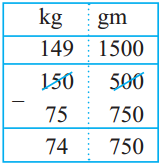74 kg 750 g of rice is left.

Example (2) A can of milk has 20 l 450 ml of milk. Another can has 18 l 800 ml. How much milk is there in the two cans altogether?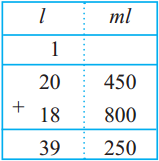The total quantity of milk is 39l 250ml.

Example (3) At a speed of 90 km per hour, what distance will a train cover in two and a half hours?

The speed of the train is 90 kmph. That is, it travels 90 km in one hour. It travels 90 more km in the second hour.
In the next half an hour, 90 ÷ 2 = 45 km
The total distance travelled is 90 + 90 + 45 = 225 km.

Example (4) If one dress requires 3 m 25 cm of cloth, how much do 4 dresses need?

Manju’s method :
3 m 25 cm for the 1st dress
+ 3 m 25 cm for the 2nd dress
+ 3 m 25 cm for the 3rd dress
3 m 25 cm for the 4th dress
_________
12 m 100 cm
1 m is 100 cm, therefore 12 + 1 = 13 m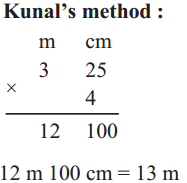Example (5)
If a wire that is 9 m 50 cm long is cut into pieces of 5 cm each, how many pieces will be made?
9 m 50 cm = (900 + 50) cm
To find out how many pieces of 5 cm can be made from a wire 950 cm long, let us use division.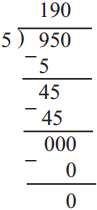Example (6) A play started at 30 minutes past 6 in the evening and finished two and three quarter hours later. What time did the play get over?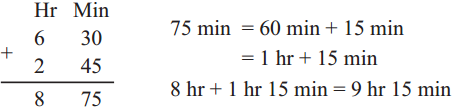The play got over at 15 minutes past 9 at night.

Note : The units for length, mass and capacity are written in decimal form. This makes it easy to carry out addition and subtraction of length, mass and capacity.

Units of measuring time are not in decimal form. It is a little more difficult to carry out additions and subtractions of those quantities.(1) 12 km 880 m + 7 km 620 m
Solution:

 km m 1 1 2 + 7 8 8 O 6 2 0 2 0 5 0 0

880m + 620 m = 1500 m
= 1km 500 m
∴ 20 km 500 m

(2) ₹ 62, 45 paise + ₹ 37, 55 paise
Solution:

 ₹ Paise 1 6 2 + 3 7 4 5 5 5 1 0 0 0 0

45 paise + 55 paise
100 paise = 1 ₹
∴ 100 rupeesSubtract the following:

(1) 15 m 15 cm – 4 m 65 cm
Solution:

 kg gm 1 4 1 1 5 1 5 – 4 1 5 6 5 1 0 5 0

We cannot subtract 65 cm from 15 cm. So, convert l m = 100 cm
∴ 10 m 50 cm

(2) 29 kg 880 gm – 8 kg 900 gm
Solution:

 kg gm 2 8 1 8 8 0 2 9 – 8 8 8 0 9 0 0 2 0 9 8 0

We cannot subtract 900 gin from 880 gm. So, convert 1 kg = 1000 gm
∴ 20 kg 980 gm